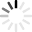Advertisement
Advertisement
Advertisement

# Pythagorean Theorem Quizzes & Trivia

c2=a2+b2. Do you remember this theorem from your maths class? Pythagoras was one of the visionaries who laid the first bricks of this theory. Take the online Pythagorean theorem quizzes and prove you are a math maverick!

Top Trending

The Pythagorean Theorem is a mathematical formula that tells the relationship between the sides in a right triangle, consisting of two legs and a hypotenuse. The Theorem is named after the ancient Greek mathematician...

Questions: 10  |  Attempts: 2138   |  Last updated: Feb 22, 2021
• Sample Question
The front of a hiking tent is shaped like a triangle. The slanted sides are both 5 feet long and the bottom of the tent is 6 feet across. What is the height of the tent in feet at the tallest point?To all the mathematics lovers out there who indulge in different formula and books for both high and low levels of study take this quiz. It is specifically designed to help you find out how much you know about the Pythagorean...

Questions: 10  |  Attempts: 381   |  Last updated: Oct 5, 2017
• Sample Question
What is the measure of the missing length?Assignment 3 (Online Quiz) Total Marks: 20 Hi my students, before you start on this quiz, ask yourself, are you ready for it? - Yes! All the way! Then hit the Start button. - No! Then please take some more time to revise...

Questions: 5  |  Attempts: 782   |  Last updated: May 16, 2018

Questions: 6  |  Attempts: 176   |  Last updated: May 8, 2013
• Sample Question
A boat left a dock and sailed 16 miles north and then sailed 16 miles east, as shown in the diagram at the right. Which of the following is a true statement about the shortest distance the boat must sail to return to the dock?Middle school math quiz to test knowledge of convers of pythagorean theorem

Questions: 8  |  Attempts: 500   |  Last updated: Jan 23, 2013
• Sample Question
A triangle with sides length of 5,12, and 13 units is a right triangle.Advertisement

AdvertisementRelated